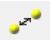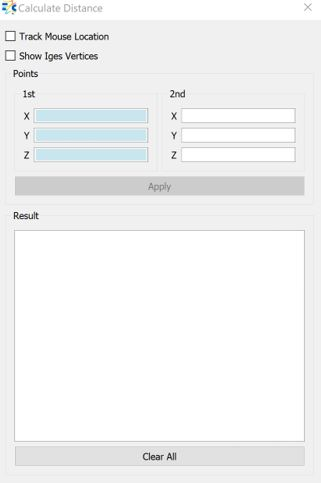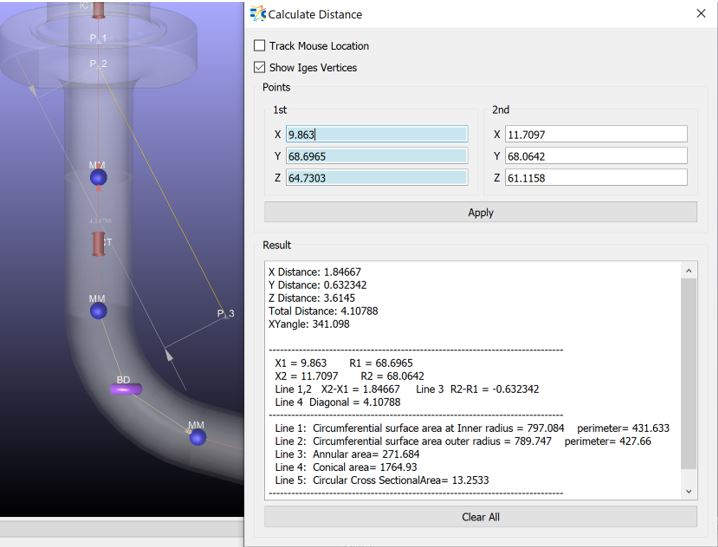# Calculate Distance

Calculate (Measure)Distance option allows the user to measure the distance between two points.There are 3 major ways to define the 2 points to be measured.

1. Track Mouse Location: tracks the mouse and gets the X, Y, Z location at the mouse clicked. The first click will set the X, Y, Z locations of the 1st point and the second click will be for 2nd point.

2. Show Iges Vertices: If there is a loaded CAD model, it is possible to select 2 of the these points to measure the distance between them. The Iges vertices can only be seen when this option is enables.

3. Write the X, Y, Z locations of the 1st and 2nd points directly.

Apply: To calculate the distance, after setting the locations of the points, the user should click on Apply.

Clear All: To clear the points’ locations and the calculations.Result section divided into 3 sections:

Section 1: Calculated Results

X Distance: Calculated difference between the X locations of 1st and 2nd points

Y Distance: Calculated difference between the Y locations of 1st and 2nd points

Z Distance: Calculated difference between the Z locations of 1st and 2nd points

Total Distance: Calculated Distance between two points

Section 2: Coordinater Informations

X1 = 1st Point’s X location

R1 = 1st Point’s Y location

X2 = 2nd Point’s X location

R2 = 2nd Point’s Y location

Section 3: Area Informations

Line 1: cylindrical area = 2*π*R1*(X2-X1)

Line 2: cylindrical area = 2*π*R2*(X2-X1)

Line 3: annular area = π*(R22-R12)

Line 4: conical area =